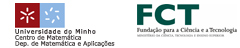EN || PT || ESJosé Espírito Santo (Universidade do Minho)Towards a canonical classical natural deduction system

This talk is about a new classical natural deduction system, presented as a typed lambda-calculus. It is designed to be isomorphic to Curien-Herbelin's calculus, both at the level of proofs and reduction, and the isomorphism is based on the correct correspondence between cut (resp. left-introduction) in sequent calculus, and substitution (resp. elimination) in natural deduction. It is a combination of Parigot's calculus with the idea of "coercion calculus" due to Cervesato-Pfenning, accommodating let-expressions in a surprising way: they expand Parigot's syntactic class of named terms.

This calculus aims to be the simultaneous answer to three problems. The first problem is the lack of a canonical natural deduction system for classical logic. The proposed calculus is not yet another classical calculus, but rather a canonical reflection in natural deduction of the impeccable treatment of classical logic by sequent calculus. The second problem is the lack of a formalization of the usual semantics of Curien-Herbelin's calculus, that explains co-terms and cuts as, respectively, contexts and hole-filling instructions. The mentioned isomorphism is the required formalization, based on the precise notions of context and hole-expression offered by the proposed calculus. The third problem is the lack of a robust process of  "read-back'' into natural deduction syntax of calculi in the sequent calculus format, that affects mainly the recent proof-theoretic efforts of derivation of  lambda-calculi for call-by-value. An isomorphic counterpart to the Q-subsystem of Curien-Herbelin's calculus is derived, obtaining a new lambda-calculus for call-by-value, combining control and let-expressions.© 3º Encontro Ibérico de Matemática :: 2010